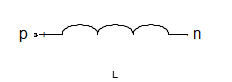# inductor

Inductor object

## Description

Use `inductor` class to create an inductor object that you can add to an existing circuit.## Creation

### Syntax

``lobj = inductor(lvalue)``
``lobj = inductor(lvalue,lname)``

### Description

example

````lobj = inductor(lvalue)` creates a inductor object, `lobj`, with a inductance of `lvalue` and default name, `L`. `lvalue` must be a numeric positive scalar.```

example

````lobj = inductor(lvalue,lname)` creates a inductor object, `lobj`, with a inductance of `lvalue` and name `lname`. `lname` must be a character vector.```

## Properties

expand all

Inductance, specified as a scalar in henries.

Example: `1e-9`

Example: `lobj.Inductance = 1e-9`

Name of inductor object, specified as a character vector. Two elements in the same circuit cannot have the same name.

Example: `'cap'`

Example: `lobj.Name = 'indcutor1'`

Names of the terminals of inductor object, specified as a cell vector These names are always `p` and `n`.

Example: `{'p' 'n'}`

Example: `lobj.Terminals = {'p' 'n'}`

Full path of the circuit to which the inductor object belongs, specified as character vector. This path appears only after the inductor is added to the circuit.

Note

"ParentPath" is only displayed after the capacitor has been added

into a circuit.

Parent nodes connected to inductor terminals, specified as a vector of integers. This property appears only after the inductor is added to a circuit.

Example: `[1 2]`

Example: `lobj.ParentNodes = [1 2]`

Note

"ParentNodes" are only displayed after the capacitor has been added

into a circuit.

## Object Functions

 `clone` Create copy of existing circuit element or circuit object

## Examples

collapse all

Create an inductor of 3e-9 henry and display the properties.

```hL1 = inductor(3e-9); disp(hL1)```
``` inductor: Inductor element Inductance: 3.0000e-09 Name: 'L' Terminals: {'p' 'n'} ```

Create an inductor object and extract the s-parameters of this inductor.

```hL = inductor(3e-9,'L3nh'); hckt = circuit('example2'); add(hckt,[1 2],hL) setports (hckt, [1 0],[2 0]) freq = linspace (1e3,2e3,100); S = sparameters(hckt,freq); disp(S)```
``` sparameters: S-parameters object NumPorts: 2 Frequencies: [100x1 double] Parameters: [2x2x100 double] Impedance: 50 rfparam(obj,i,j) returns S-parameter Sij ```

Add an inductor to a circuit, display the parent path and parent nodes.

```hL = inductor(3e-9,'L3n9'); hckt = circuit('example3'); add(hckt,[1 2],hL) setports(hckt, [1 0],[2 0]) disp(hL)```
``` inductor: Inductor element Inductance: 3.0000e-09 Name: 'L3n9' Terminals: {'p' 'n'} ParentNodes: [1 2] ParentPath: 'example3' ```